SPSS TUTORIALS BASICS ANOVA REGRESSION FACTOR CORRELATION

# SPSS String Variables Basics

For working proficiently with SPSS string variables , it greatly helps to understand some string basics. This tutorial explains what SPSS string variables are and demonstrates their main properties.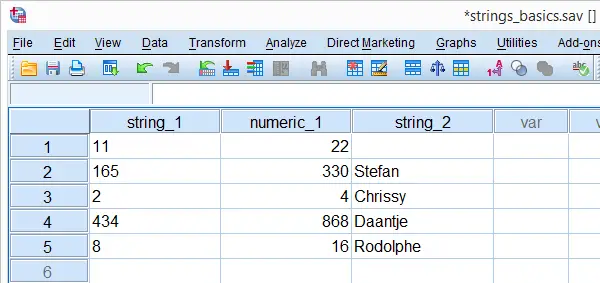## SPSS String Variables - What Are They?

String variables are one of SPSS' two variable types. What really defines a string variable is the way its values are stored internally.We won't go into this technical matter here but those who really want to know may consult our Unicode tutorial. A simpler definition is that string variables are variables that hold zero or more text characters.
String values are always treated as text, even if they contain only numbers. Some surprising consequences of this are shown towards the end of this tutorial.

## SPSS String Format

String variables in SPSS usually have an “A” format, where “A” denotes “Alphanumeric”. This can be seen by running the following line of syntax display dictionary. after opening the data. The result, shown in the screenshot below, confirms that we have two string variables having A3 and A8 formats.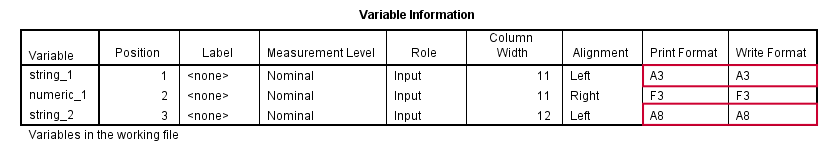The numeric suffixes (3 and 8 here) are the numbers of bytes that the values can hold. Starting from SPSS version 16, some characters may consist of two bytes.This is explained in Unicode mode. If you don't want to go into details, just choose string lengths that are twice the number of characters they need to contain to stay on the safe side.

## SPSS String Command

Commands that pass values into variables, most notably `COMPUTE` and IF, can be used for both existing and new numeric variables. However, they can't be used for new string variables; you must first create one or more new, empty string variables before you can pass values into them. This is done with the `STRING` command. Its most basic use is STRING variable_names (A10). As explained earlier, A10 means that the new variable can hold values of up to 10 bytes. The syntax below creates a new string variable in our test data.

*1. Create empty new string variable with string command.

string string_3(a10).

*2. Pass values into new string variable.

compute string_3 = 'Hello'.
exe.

## SPSS String Function

SPSS' string function converts numeric values to string values. Its most basic use is compute s2 = string(s1,f1). where s2 is a string variable, s1 is a numeric variable or value and f1 is the numeric format to be used.
With regard to our test data, the syntax below shows how to convert numeric_1 into (previously created) string_3. In order to capture all three digits, we need to specify f3 as the format.

*Convert numeric_1 to (existing) string variable with string function.

compute string_3 = string(numeric_1,f3).
exe.

## Quotes Around String Values

If you use string values in syntax, put quotes around them. For example, say we want to flag all cases whose name is “Stefan”. The screenshot shows the desired result. The syntax below demonstrates the wrong way and then the right way to do so.A faster way to do this is `compute find_stefan = string_2 = 'Stefan'.` Compute A = B = C explains how this works.

*1. Compute empty flag variable.

compute find_stefan = 0.
exe.

*2. Wrong way: without quotes Stefan is thought to be variable name.

if string_2 = Stefan find_stefan = 1.
exe.

*3. Right way: quotes around Stefan.

if string_2 = 'Stefan' find_stefan = 1.
exe.

## Result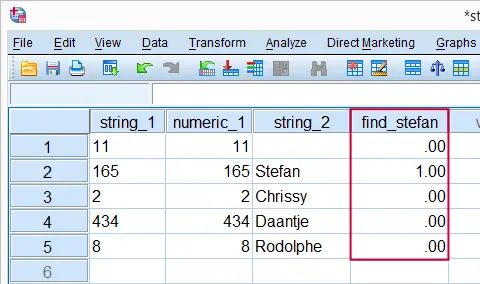Flagging Cases Whose Name is Stefan

Note that the second step triggers SPSS error #4285: due to the omitted quotes, SPSS thinks that Stefan refers to a variable name and doesn't find it in the data.

## String Values are Case Sensitive

Now let's create a similar flag variable for cases called “Chrissy”. After running step 2 in the syntax below, you can see in data view that no cases have been flagged; it uses the wrong casing. Step 3, using the correct casing, does flag “Chrissy” correctly.

*1. Compute empty flag variable.

compute find_chrissy = 0.
exe.

*2. Line below doesn't flag any cases because 'chrissy' is not the same as 'Chrissy'.

if string_2 = 'chrissy' find_chrissy = 1.
exe.

*3. Right way: 'Chrissy' instead of 'chrissy'.

if string_2 = 'Chrissy' find_chrissy = 1.
exe.

## SPSS String Variables - System Missing Values

There's no such thing as a system missing value in a string variable; string values consisting of zero characters which are called empty strings are valid values in SPSS.Also note that you don't see a dot (indicating a system missing value) in an empty cell of a string variable. We can confirm this by running FREQUENCIES: frequencies string_2. Note that the empty string value is among the valid values.

## Result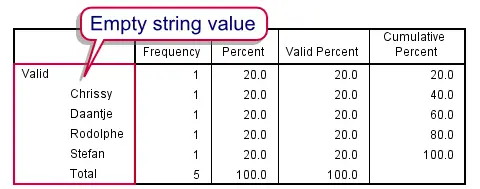## User Missing Values in String Variables

Over the years, we've seen many forum questions (and some heated debates) regarding user missing values in string variables. Well, running missing values string_2(''). specifies the empty string as a user missing value. This can be confirmed by rerunning its frequency table; the empty string is now in the missing values section as shown by the screenshot.

## Result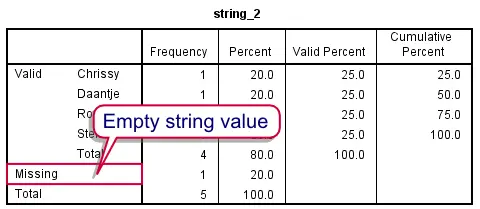## Sorting on String Variables

String values are seen as text, even if they consist of only numbers. A consequence is that string values are sorted alphabetically. To see what this means, run sort cases by string_1.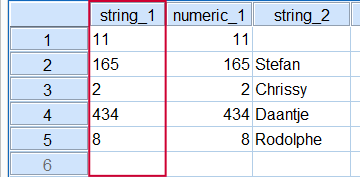Alphabetical Sorting of string_1

If this result puzzles you, represent the numbers 0 through 9 by letters a through j. Clearly, “bb” (= 11) comes before “c” (= 2) if sorted alphabetically.

## No Calculations on String Variables

Because string values are seen as text, you can't do any calculations on them. For instance a COMPUTE command with some numeric function like compute string_1 = string_1 * 2. will trigger SPSS error #4307. It basically tries to tell us that our command crashed because a string variable was used in a calculation.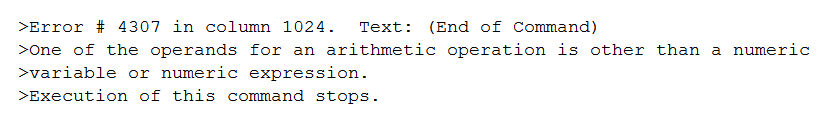In a similar vein, most procedures involve calculations and thus won't run on string variables either. For example, descriptives string_1. won't produce any other results than a warning that the command crashed because only string variables were involved.

# Tell us what you think!

*Required field. Your comment will show up after approval from a moderator.

# THIS TUTORIAL HAS 11 COMMENTS:

•### By Michelle on June 22nd, 2023

Thank-you! It worked :)